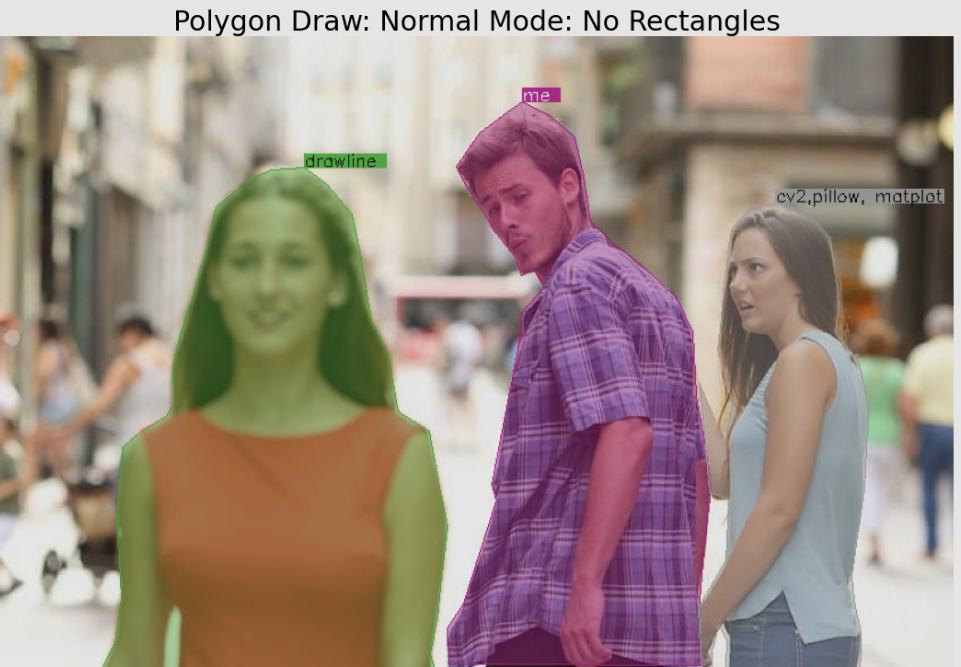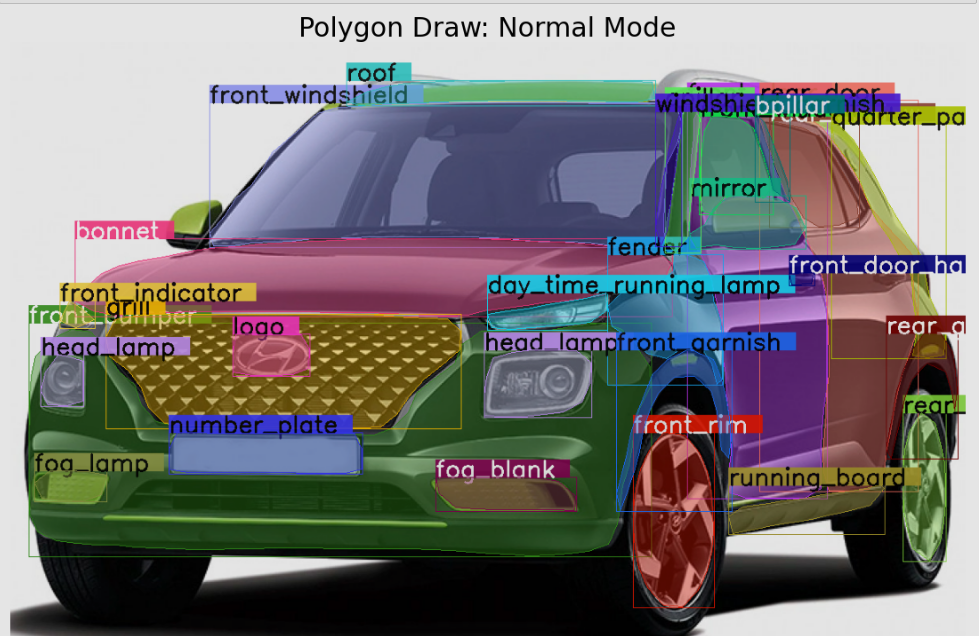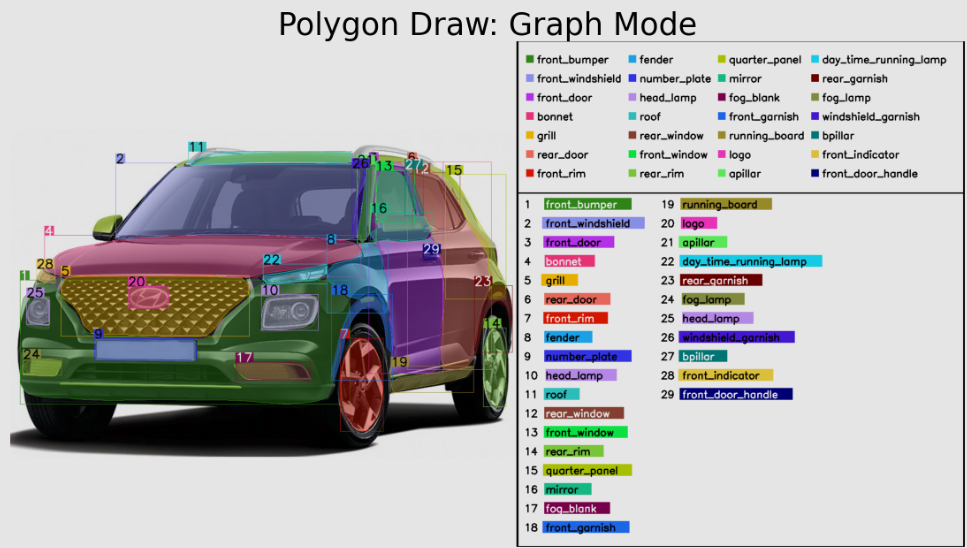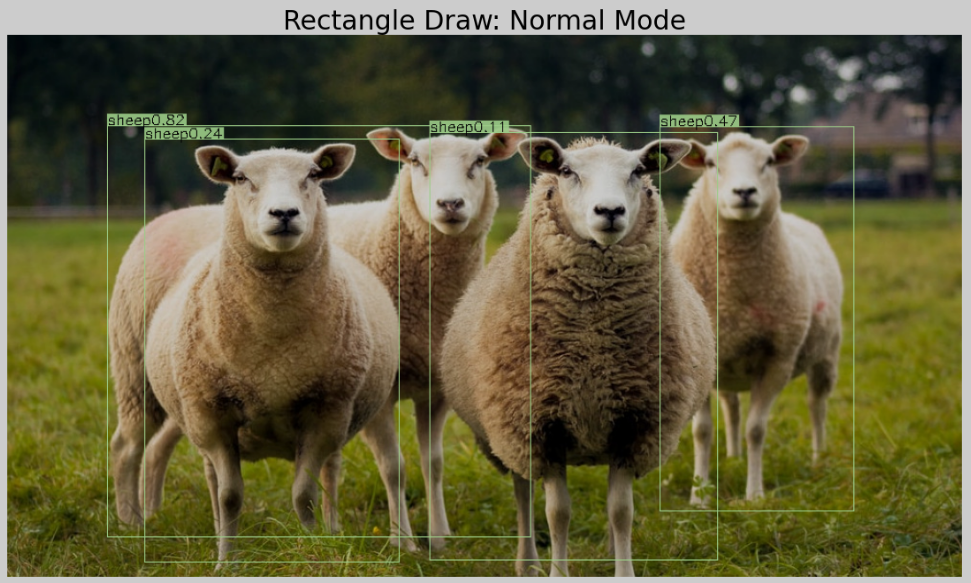Clean draw of rectangle and polygon contours

# Drawline

Say goodbye to endless searching and trial-and-error with image overlays! Drawline makes it effortless to overlay polyggon contours, rectangles and even auto-handles label colors, font size and line thickness. With the option to override, Drawline takes the hassle out of image drawing customization

### Install

pip install drawline


### Screenshots

1. Polygon draw (Normal mode): Good to use when there are less number of polygons to draw.# Go to 'How to Use' section for detail code
result_image = draw_poly(image, {YOUR_CONTOURS}, label={YOUR_LABELS}, graph_mode=False, show_rect=False)


1. Polygon draw (Graph mode): Good to use when there are many number of polygons to draw. Overcomes the overlay labels problem.# Go to 'How to Use' section for detail code
result_image = draw_poly(image, {YOUR_CONTOURS}, label={YOUR_LABELS}, graph_mode=True)


1. Rectangle draw (normal mode): You can also simlpy draw a rectangle instead of polygon.# Go to 'How to Use' section for detail code
result_image = draw_rect(image, {YOUR_RECTANGLE_COORDINATES}, label={YOUR_LABELS})


## How to use

Checkout the example: Notebook Example (NB viewer) or Github Viewer

OR

from drawline import draw_poly, draw_rect
import cv2

img_pth = '/PATH/TO/IMAGE.jpg'

# Single  Rectangle example
result_image = draw_rect(image, [XMIN, YMIN, XMAX, YMAX], labels='label_1')

# Multiple Rectangles example
result_image = draw_rect(image,
[[XMIN_1, YMIN_1, XMAX_1, YMAX_1],
[XMIN_2, YMIN_2, XMAX_2, YMAX_2]],
labels=['Label_1', 'Label_2'])

# Single Contours Polygon example
result_image = draw_poly(image, CONTOUR, label='label_1')

# Multiple Contours Polygon points
result_image = draw_poly(image, CONTOURS, label=['label_1', 'label_2', ...])

# Assigning Labels is optional, if not given no label name will be displayed


### Default options

def draw_rect(image, points, rgb=None, label_transparency=0.1, thickness=None, labels=None,
label_rgb=None, label_bg_rgb=None, label_font_size=None,
random_color=False, graph_mode=False):
"""
Draws rectangle from given coordinates
:param image: (Numpy) numpy matrix image
:param points: (List) List of rectangle coordinates: [[xmin, ymin, xmax, ymax]]
:param rgb: (Tuple) RGB values: (R, G, B)
:param label_transparency: (float) transparency for the labels
:param thickness: (Integer) of line in px: eg: 2
:param labels: (List) list of strings: []
:param label_rgb: (Tuple) RGB text color for labels: (R,G,B)
:param label_bg_rgb: (Tuple) RGB label background color: (R,G,B)
:param label_font_size: (Integer) Font size of label in px: 2
:param random_color: (Boolean) pick random colors for lines.
:param graph_mode: (Boolean) Writes labels to a border instead in the image itself (Good to use when to many boxes
obstructing the view)

:return: (numpy) drawn rectangles on image
"""

def draw_poly(image, contours, fill_in=True, label_transparency=0.1, fill_transparency=0.4, rgb=None, thickness=None,
show_rect=True, labels=None, label_rgb=None, label_bg_rgb=None, label_font_size=None, random_color=False,
graph_mode=False):
"""
Draws polygon and fills in color from given contours
:param image: (Numpy) numpy matrix image
:param contours: (List) of contours
:param fill_in: (Boolean) fill color inside the polygon.
:param label_transparency: (float) transparency for the labels
:param fill_transparency: (Float) transparency of fill_in color.
:param rgb: RGB values: (Tuple) rgb color of line and polyfgon (R, G, B)
:param thickness: (Int) Thickness of line
:param show_rect: (Boolean) Show rectangle
:param labels: (List of strings) List of label names
:param label_rgb: (Tuple) RGB color of labels
:param label_bg_rgb: (Tuple) RGB color of Label background
:param label_font_size: (Int) Label font size
:param random_color: (Boolean) Randomize RGB color
:param graph_mode: (Boolean) Writes labels to a border instead in the image itself (Good to use when to many boxes
obstructing the view)

:return: (Numpy) drawn polygon on image
"""


## Project details

This version0.3.40.3.30.3.10.2.20.1.40.0.20.0.1

Uploaded source
Uploaded py3